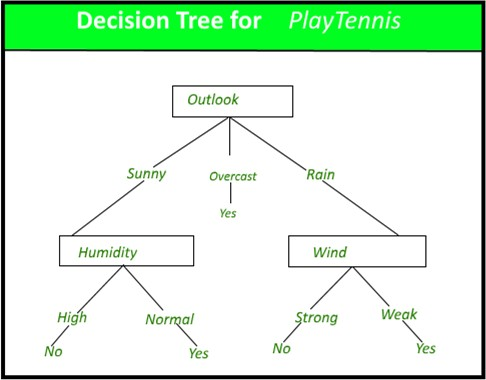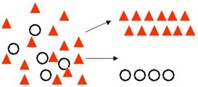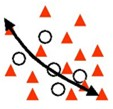Earn 20 XP

### What is a Decision Tree?

A decision tree is the most powerful and popular tool for classification and prediction.

A Decision tree is a flowchart-like tree structure where:

• each internal node denotes a test on an attribute/feature,
• each branch represents an outcome of the test, and
• each leaf node (terminal node) holds a class label (Yes and No in this case).### Decision Trees

Regression tree analysis is used when the predicted outcome can be considered a real number (e.g., the price of a house or a patient's length of stay in a hospital).

### CART

You might encounter the term 'CART' while building ML models. It's nothing new but the same old Decision Tree since it can be used for both Classification and Regression.

CART = Classification and Regression Trees, an umbrella term for:

Classification Trees: where the target variable is categorical, the tree is used to identify the "class" within which a target variable would likely fall into.Regression Trees: where the target variable is continuous, and the tree is used to predict its value.